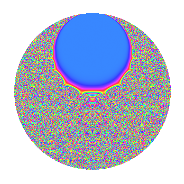# Properties

 Label 2.34.a.bLevel 2 Weight 34 Character orbit 2.a Self dual Yes Analytic conductor 13.797 Analytic rank 0 Dimension 2 CM No Inner twists 1

# Related objects

## Newspace parameters

 Level: $$N$$ = $$2$$ Weight: $$k$$ = $$34$$ Character orbit: $$[\chi]$$ = 2.a (trivial)

## Newform invariants

 Self dual: Yes Analytic conductor: $$13.7965657762$$ Analytic rank: $$0$$ Dimension: $$2$$ Coefficient field: $$\mathbb{Q}[x]/(x^{2} - \cdots)$$ Coefficient ring: $$\Z[a_1, a_2, a_3]$$ Coefficient ring index: $$2^{7}\cdot 3\cdot 5\cdot 11$$ Fricke sign: $$-1$$ Sato-Tate group: $\mathrm{SU}(2)$

## $q$-expansion

Coefficients of the $$q$$-expansion are expressed in terms of $$\beta = 10560\sqrt{79829689}$$. We also show the integral $$q$$-expansion of the trace form.

 $$f(q)$$ $$=$$ $$q$$ $$+ 65536 q^{2}$$ $$+ ( 4178244 - \beta ) q^{3}$$ $$+ 4294967296 q^{4}$$ $$+ ( -2666238330 - 3996 \beta ) q^{5}$$ $$+ ( 273825398784 - 65536 \beta ) q^{6}$$ $$+ ( 66359547937928 + 896238 \beta ) q^{7}$$ $$+ 281474976710656 q^{8}$$ $$+ ( 3360493163638413 - 8356488 \beta ) q^{9}$$ $$+O(q^{10})$$ $$q$$ $$+65536 q^{2}$$ $$+(4178244 - \beta) q^{3}$$ $$+4294967296 q^{4}$$ $$+(-2666238330 - 3996 \beta) q^{5}$$ $$+(273825398784 - 65536 \beta) q^{6}$$ $$+(66359547937928 + 896238 \beta) q^{7}$$ $$+281474976710656 q^{8}$$ $$+(3360493163638413 - 8356488 \beta) q^{9}$$ $$+(-174734595194880 - 261881856 \beta) q^{10}$$ $$+(-79052735711361588 + 2213453277 \beta) q^{11}$$ $$+(17945421334708224 - 4294967296 \beta) q^{12}$$ $$+(2491699257749546894 - 13597194492 \beta) q^{13}$$ $$+(4348939333660049408 + 58735853568 \beta) q^{14}$$ $$+(35561635450747625880 - 14030024694 \beta) q^{15}$$ $$+18446744073709551616 q^{16}$$ $$+($$$$15\!\cdots\!38$$$$- 833438065608 \beta) q^{17}$$ $$+($$$$22\!\cdots\!68$$$$- 547650797568 \beta) q^{18}$$ $$+($$$$11\!\cdots\!00$$$$+ 13790479962267 \beta) q^{19}$$ $$+(-11451406430691655680 - 17162689314816 \beta) q^{20}$$ $$+(-$$$$77\!\cdots\!68$$$$- 62614846891856 \beta) q^{21}$$ $$+(-$$$$51\!\cdots\!68$$$$+ 145060873961472 \beta) q^{22}$$ $$+(-$$$$35\!\cdots\!76$$$$+ 63128735356554 \beta) q^{23}$$ $$+($$$$11\!\cdots\!64$$$$- 281474976710656 \beta) q^{24}$$ $$+($$$$25\!\cdots\!75$$$$+ 21308576733360 \beta) q^{25}$$ $$+($$$$16\!\cdots\!84$$$$- 891105738227712 \beta) q^{26}$$ $$+($$$$65\!\cdots\!60$$$$+ 2163651957070038 \beta) q^{27}$$ $$+($$$$28\!\cdots\!88$$$$+ 3849312899432448 \beta) q^{28}$$ $$+(-$$$$12\!\cdots\!10$$$$- 10405860886172556 \beta) q^{29}$$ $$+($$$$23\!\cdots\!80$$$$- 919471698345984 \beta) q^{30}$$ $$+(-$$$$21\!\cdots\!48$$$$+ 2188390162942584 \beta) q^{31}$$ $$+$$$$12\!\cdots\!76$$$$q^{32}$$ $$+(-$$$$20\!\cdots\!72$$$$+ 88301083585267176 \beta) q^{33}$$ $$+($$$$99\!\cdots\!68$$$$- 54620197067685888 \beta) q^{34}$$ $$+(-$$$$32\!\cdots\!40$$$$- 267562337668362828 \beta) q^{35}$$ $$+($$$$14\!\cdots\!48$$$$- 35890842669416448 \beta) q^{36}$$ $$+($$$$58\!\cdots\!38$$$$+ 704679249787856532 \beta) q^{37}$$ $$+($$$$73\!\cdots\!00$$$$+ 903772894807130112 \beta) q^{38}$$ $$+($$$$13\!\cdots\!36$$$$- 2548511654052578942 \beta) q^{39}$$ $$+(-$$$$75\!\cdots\!80$$$$- 1124774006935781376 \beta) q^{40}$$ $$+(-$$$$83\!\cdots\!38$$$$+ 5060866057679897136 \beta) q^{41}$$ $$+(-$$$$50\!\cdots\!48$$$$- 4103526605904674816 \beta) q^{42}$$ $$+(-$$$$18\!\cdots\!76$$$$+ 795637191126404277 \beta) q^{43}$$ $$+(-$$$$33\!\cdots\!48$$$$+ 9506709435939028992 \beta) q^{44}$$ $$+($$$$28\!\cdots\!10$$$$- 13406250293289313308 \beta) q^{45}$$ $$+(-$$$$23\!\cdots\!36$$$$+ 4137204800327122944 \beta) q^{46}$$ $$+($$$$14\!\cdots\!28$$$$- 18059171324272980012 \beta) q^{47}$$ $$+($$$$77\!\cdots\!04$$$$- 18446744073709551616 \beta) q^{48}$$ $$+($$$$38\!\cdots\!77$$$$+$$$$11\!\cdots\!28$$$$\beta) q^{49}$$ $$+($$$$16\!\cdots\!00$$$$+ 1396478884797480960 \beta) q^{50}$$ $$+($$$$80\!\cdots\!72$$$$-$$$$15\!\cdots\!90$$$$\beta) q^{51}$$ $$+($$$$10\!\cdots\!24$$$$- 58399505660491333632 \beta) q^{52}$$ $$+(-$$$$21\!\cdots\!46$$$$+ 12899519303831752788 \beta) q^{53}$$ $$+($$$$42\!\cdots\!60$$$$+$$$$14\!\cdots\!68$$$$\beta) q^{54}$$ $$+(-$$$$78\!\cdots\!60$$$$+$$$$30\!\cdots\!38$$$$\beta) q^{55}$$ $$+($$$$18\!\cdots\!68$$$$+$$$$25\!\cdots\!28$$$$\beta) q^{56}$$ $$+(-$$$$11\!\cdots\!00$$$$-$$$$10\!\cdots\!52$$$$\beta) q^{57}$$ $$+(-$$$$82\!\cdots\!60$$$$-$$$$68\!\cdots\!16$$$$\beta) q^{58}$$ $$+($$$$93\!\cdots\!80$$$$+$$$$15\!\cdots\!73$$$$\beta) q^{59}$$ $$+($$$$15\!\cdots\!80$$$$- 60258497222802407424 \beta) q^{60}$$ $$+($$$$23\!\cdots\!02$$$$-$$$$42\!\cdots\!92$$$$\beta) q^{61}$$ $$+(-$$$$14\!\cdots\!28$$$$+$$$$14\!\cdots\!24$$$$\beta) q^{62}$$ $$+($$$$15\!\cdots\!64$$$$+$$$$24\!\cdots\!30$$$$\beta) q^{63}$$ $$+$$$$79\!\cdots\!36$$$$q^{64}$$ $$+($$$$47\!\cdots\!80$$$$-$$$$99\!\cdots\!64$$$$\beta) q^{65}$$ $$+(-$$$$13\!\cdots\!92$$$$+$$$$57\!\cdots\!36$$$$\beta) q^{66}$$ $$+(-$$$$37\!\cdots\!32$$$$-$$$$98\!\cdots\!21$$$$\beta) q^{67}$$ $$+($$$$64\!\cdots\!48$$$$-$$$$35\!\cdots\!68$$$$\beta) q^{68}$$ $$+(-$$$$71\!\cdots\!44$$$$+$$$$35\!\cdots\!52$$$$\beta) q^{69}$$ $$+(-$$$$21\!\cdots\!40$$$$-$$$$17\!\cdots\!08$$$$\beta) q^{70}$$ $$+($$$$13\!\cdots\!32$$$$-$$$$32\!\cdots\!62$$$$\beta) q^{71}$$ $$+($$$$94\!\cdots\!28$$$$-$$$$23\!\cdots\!28$$$$\beta) q^{72}$$ $$+(-$$$$26\!\cdots\!66$$$$-$$$$70\!\cdots\!72$$$$\beta) q^{73}$$ $$+($$$$38\!\cdots\!68$$$$+$$$$46\!\cdots\!52$$$$\beta) q^{74}$$ $$+(-$$$$82\!\cdots\!00$$$$-$$$$25\!\cdots\!35$$$$\beta) q^{75}$$ $$+($$$$48\!\cdots\!00$$$$+$$$$59\!\cdots\!32$$$$\beta) q^{76}$$ $$+($$$$12\!\cdots\!36$$$$+$$$$76\!\cdots\!12$$$$\beta) q^{77}$$ $$+($$$$86\!\cdots\!96$$$$-$$$$16\!\cdots\!12$$$$\beta) q^{78}$$ $$+(-$$$$13\!\cdots\!40$$$$-$$$$55\!\cdots\!68$$$$\beta) q^{79}$$ $$+(-$$$$49\!\cdots\!80$$$$-$$$$73\!\cdots\!36$$$$\beta) q^{80}$$ $$+(-$$$$37\!\cdots\!59$$$$-$$$$97\!\cdots\!64$$$$\beta) q^{81}$$ $$+(-$$$$54\!\cdots\!68$$$$+$$$$33\!\cdots\!96$$$$\beta) q^{82}$$ $$+(-$$$$18\!\cdots\!96$$$$+$$$$35\!\cdots\!35$$$$\beta) q^{83}$$ $$+(-$$$$33\!\cdots\!28$$$$-$$$$26\!\cdots\!76$$$$\beta) q^{84}$$ $$+($$$$29\!\cdots\!60$$$$-$$$$60\!\cdots\!08$$$$\beta) q^{85}$$ $$+(-$$$$12\!\cdots\!36$$$$+$$$$52\!\cdots\!72$$$$\beta) q^{86}$$ $$+($$$$92\!\cdots\!60$$$$+$$$$82\!\cdots\!46$$$$\beta) q^{87}$$ $$+(-$$$$22\!\cdots\!28$$$$+$$$$62\!\cdots\!12$$$$\beta) q^{88}$$ $$+($$$$15\!\cdots\!90$$$$-$$$$50\!\cdots\!40$$$$\beta) q^{89}$$ $$+($$$$18\!\cdots\!60$$$$-$$$$87\!\cdots\!88$$$$\beta) q^{90}$$ $$+($$$$56\!\cdots\!32$$$$+$$$$13\!\cdots\!96$$$$\beta) q^{91}$$ $$+(-$$$$15\!\cdots\!96$$$$+$$$$27\!\cdots\!84$$$$\beta) q^{92}$$ $$+(-$$$$28\!\cdots\!12$$$$+$$$$21\!\cdots\!44$$$$\beta) q^{93}$$ $$+($$$$94\!\cdots\!08$$$$-$$$$11\!\cdots\!32$$$$\beta) q^{94}$$ $$+(-$$$$49\!\cdots\!00$$$$-$$$$45\!\cdots\!10$$$$\beta) q^{95}$$ $$+($$$$50\!\cdots\!44$$$$-$$$$12\!\cdots\!76$$$$\beta) q^{96}$$ $$+($$$$34\!\cdots\!58$$$$-$$$$24\!\cdots\!20$$$$\beta) q^{97}$$ $$+($$$$25\!\cdots\!72$$$$+$$$$77\!\cdots\!08$$$$\beta) q^{98}$$ $$+(-$$$$43\!\cdots\!44$$$$+$$$$80\!\cdots\!45$$$$\beta) q^{99}$$ $$+O(q^{100})$$ $$\operatorname{Tr}(f)(q)$$ $$=$$ $$2q$$ $$\mathstrut +\mathstrut 131072q^{2}$$ $$\mathstrut +\mathstrut 8356488q^{3}$$ $$\mathstrut +\mathstrut 8589934592q^{4}$$ $$\mathstrut -\mathstrut 5332476660q^{5}$$ $$\mathstrut +\mathstrut 547650797568q^{6}$$ $$\mathstrut +\mathstrut 132719095875856q^{7}$$ $$\mathstrut +\mathstrut 562949953421312q^{8}$$ $$\mathstrut +\mathstrut 6720986327276826q^{9}$$ $$\mathstrut +\mathstrut O(q^{10})$$ $$2q$$ $$\mathstrut +\mathstrut 131072q^{2}$$ $$\mathstrut +\mathstrut 8356488q^{3}$$ $$\mathstrut +\mathstrut 8589934592q^{4}$$ $$\mathstrut -\mathstrut 5332476660q^{5}$$ $$\mathstrut +\mathstrut 547650797568q^{6}$$ $$\mathstrut +\mathstrut 132719095875856q^{7}$$ $$\mathstrut +\mathstrut 562949953421312q^{8}$$ $$\mathstrut +\mathstrut 6720986327276826q^{9}$$ $$\mathstrut -\mathstrut 349469190389760q^{10}$$ $$\mathstrut -\mathstrut 158105471422723176q^{11}$$ $$\mathstrut +\mathstrut 35890842669416448q^{12}$$ $$\mathstrut +\mathstrut 4983398515499093788q^{13}$$ $$\mathstrut +\mathstrut 8697878667320098816q^{14}$$ $$\mathstrut +\mathstrut 71123270901495251760q^{15}$$ $$\mathstrut +\mathstrut 36893488147419103232q^{16}$$ $$\mathstrut +\mathstrut$$$$30\!\cdots\!76$$$$q^{17}$$ $$\mathstrut +\mathstrut$$$$44\!\cdots\!36$$$$q^{18}$$ $$\mathstrut +\mathstrut$$$$22\!\cdots\!00$$$$q^{19}$$ $$\mathstrut -\mathstrut 22902812861383311360q^{20}$$ $$\mathstrut -\mathstrut$$$$15\!\cdots\!36$$$$q^{21}$$ $$\mathstrut -\mathstrut$$$$10\!\cdots\!36$$$$q^{22}$$ $$\mathstrut -\mathstrut$$$$71\!\cdots\!52$$$$q^{23}$$ $$\mathstrut +\mathstrut$$$$23\!\cdots\!28$$$$q^{24}$$ $$\mathstrut +\mathstrut$$$$51\!\cdots\!50$$$$q^{25}$$ $$\mathstrut +\mathstrut$$$$32\!\cdots\!68$$$$q^{26}$$ $$\mathstrut +\mathstrut$$$$13\!\cdots\!20$$$$q^{27}$$ $$\mathstrut +\mathstrut$$$$57\!\cdots\!76$$$$q^{28}$$ $$\mathstrut -\mathstrut$$$$25\!\cdots\!20$$$$q^{29}$$ $$\mathstrut +\mathstrut$$$$46\!\cdots\!60$$$$q^{30}$$ $$\mathstrut -\mathstrut$$$$43\!\cdots\!96$$$$q^{31}$$ $$\mathstrut +\mathstrut$$$$24\!\cdots\!52$$$$q^{32}$$ $$\mathstrut -\mathstrut$$$$40\!\cdots\!44$$$$q^{33}$$ $$\mathstrut +\mathstrut$$$$19\!\cdots\!36$$$$q^{34}$$ $$\mathstrut -\mathstrut$$$$64\!\cdots\!80$$$$q^{35}$$ $$\mathstrut +\mathstrut$$$$28\!\cdots\!96$$$$q^{36}$$ $$\mathstrut +\mathstrut$$$$11\!\cdots\!76$$$$q^{37}$$ $$\mathstrut +\mathstrut$$$$14\!\cdots\!00$$$$q^{38}$$ $$\mathstrut +\mathstrut$$$$26\!\cdots\!72$$$$q^{39}$$ $$\mathstrut -\mathstrut$$$$15\!\cdots\!60$$$$q^{40}$$ $$\mathstrut -\mathstrut$$$$16\!\cdots\!76$$$$q^{41}$$ $$\mathstrut -\mathstrut$$$$10\!\cdots\!96$$$$q^{42}$$ $$\mathstrut -\mathstrut$$$$36\!\cdots\!52$$$$q^{43}$$ $$\mathstrut -\mathstrut$$$$67\!\cdots\!96$$$$q^{44}$$ $$\mathstrut +\mathstrut$$$$57\!\cdots\!20$$$$q^{45}$$ $$\mathstrut -\mathstrut$$$$46\!\cdots\!72$$$$q^{46}$$ $$\mathstrut +\mathstrut$$$$28\!\cdots\!56$$$$q^{47}$$ $$\mathstrut +\mathstrut$$$$15\!\cdots\!08$$$$q^{48}$$ $$\mathstrut +\mathstrut$$$$76\!\cdots\!54$$$$q^{49}$$ $$\mathstrut +\mathstrut$$$$33\!\cdots\!00$$$$q^{50}$$ $$\mathstrut +\mathstrut$$$$16\!\cdots\!44$$$$q^{51}$$ $$\mathstrut +\mathstrut$$$$21\!\cdots\!48$$$$q^{52}$$ $$\mathstrut -\mathstrut$$$$43\!\cdots\!92$$$$q^{53}$$ $$\mathstrut +\mathstrut$$$$85\!\cdots\!20$$$$q^{54}$$ $$\mathstrut -\mathstrut$$$$15\!\cdots\!20$$$$q^{55}$$ $$\mathstrut +\mathstrut$$$$37\!\cdots\!36$$$$q^{56}$$ $$\mathstrut -\mathstrut$$$$23\!\cdots\!00$$$$q^{57}$$ $$\mathstrut -\mathstrut$$$$16\!\cdots\!20$$$$q^{58}$$ $$\mathstrut +\mathstrut$$$$18\!\cdots\!60$$$$q^{59}$$ $$\mathstrut +\mathstrut$$$$30\!\cdots\!60$$$$q^{60}$$ $$\mathstrut +\mathstrut$$$$46\!\cdots\!04$$$$q^{61}$$ $$\mathstrut -\mathstrut$$$$28\!\cdots\!56$$$$q^{62}$$ $$\mathstrut +\mathstrut$$$$31\!\cdots\!28$$$$q^{63}$$ $$\mathstrut +\mathstrut$$$$15\!\cdots\!72$$$$q^{64}$$ $$\mathstrut +\mathstrut$$$$95\!\cdots\!60$$$$q^{65}$$ $$\mathstrut -\mathstrut$$$$26\!\cdots\!84$$$$q^{66}$$ $$\mathstrut -\mathstrut$$$$74\!\cdots\!64$$$$q^{67}$$ $$\mathstrut +\mathstrut$$$$12\!\cdots\!96$$$$q^{68}$$ $$\mathstrut -\mathstrut$$$$14\!\cdots\!88$$$$q^{69}$$ $$\mathstrut -\mathstrut$$$$42\!\cdots\!80$$$$q^{70}$$ $$\mathstrut +\mathstrut$$$$26\!\cdots\!64$$$$q^{71}$$ $$\mathstrut +\mathstrut$$$$18\!\cdots\!56$$$$q^{72}$$ $$\mathstrut -\mathstrut$$$$52\!\cdots\!32$$$$q^{73}$$ $$\mathstrut +\mathstrut$$$$76\!\cdots\!36$$$$q^{74}$$ $$\mathstrut -\mathstrut$$$$16\!\cdots\!00$$$$q^{75}$$ $$\mathstrut +\mathstrut$$$$96\!\cdots\!00$$$$q^{76}$$ $$\mathstrut +\mathstrut$$$$24\!\cdots\!72$$$$q^{77}$$ $$\mathstrut +\mathstrut$$$$17\!\cdots\!92$$$$q^{78}$$ $$\mathstrut -\mathstrut$$$$26\!\cdots\!80$$$$q^{79}$$ $$\mathstrut -\mathstrut$$$$98\!\cdots\!60$$$$q^{80}$$ $$\mathstrut -\mathstrut$$$$75\!\cdots\!18$$$$q^{81}$$ $$\mathstrut -\mathstrut$$$$10\!\cdots\!36$$$$q^{82}$$ $$\mathstrut -\mathstrut$$$$37\!\cdots\!92$$$$q^{83}$$ $$\mathstrut -\mathstrut$$$$66\!\cdots\!56$$$$q^{84}$$ $$\mathstrut +\mathstrut$$$$58\!\cdots\!20$$$$q^{85}$$ $$\mathstrut -\mathstrut$$$$24\!\cdots\!72$$$$q^{86}$$ $$\mathstrut +\mathstrut$$$$18\!\cdots\!20$$$$q^{87}$$ $$\mathstrut -\mathstrut$$$$44\!\cdots\!56$$$$q^{88}$$ $$\mathstrut +\mathstrut$$$$30\!\cdots\!80$$$$q^{89}$$ $$\mathstrut +\mathstrut$$$$37\!\cdots\!20$$$$q^{90}$$ $$\mathstrut +\mathstrut$$$$11\!\cdots\!64$$$$q^{91}$$ $$\mathstrut -\mathstrut$$$$30\!\cdots\!92$$$$q^{92}$$ $$\mathstrut -\mathstrut$$$$57\!\cdots\!24$$$$q^{93}$$ $$\mathstrut +\mathstrut$$$$18\!\cdots\!16$$$$q^{94}$$ $$\mathstrut -\mathstrut$$$$98\!\cdots\!00$$$$q^{95}$$ $$\mathstrut +\mathstrut$$$$10\!\cdots\!88$$$$q^{96}$$ $$\mathstrut +\mathstrut$$$$68\!\cdots\!16$$$$q^{97}$$ $$\mathstrut +\mathstrut$$$$50\!\cdots\!44$$$$q^{98}$$ $$\mathstrut -\mathstrut$$$$86\!\cdots\!88$$$$q^{99}$$ $$\mathstrut +\mathstrut O(q^{100})$$

## Embeddings

For each embedding $$\iota_m$$ of the coefficient field, the values $$\iota_m(a_n)$$ are shown below.

For more information on an embedded modular form you can click on its label.

Label $$\iota_m(\nu)$$ $$a_{2}$$ $$a_{3}$$ $$a_{4}$$ $$a_{5}$$ $$a_{6}$$ $$a_{7}$$ $$a_{8}$$ $$a_{9}$$ $$a_{10}$$
1.1
 4467.87 −4466.87
65536.0 −9.01727e7 4.29497e9 −3.79693e11 −5.90956e12 1.50920e14 2.81475e14 2.57205e15 −2.48835e16
1.2 65536.0 9.85292e7 4.29497e9 3.74360e11 6.45721e12 −1.82013e13 2.81475e14 4.14894e15 2.45341e16
 $$n$$: e.g. 2-40 or 990-1000 Significant digits: Format: Complex embeddings Normalized embeddings Satake parameters Satake angles

## Inner twists

This newform does not admit any (nontrivial) inner twists.

## Atkin-Lehner signs

$$p$$ Sign
$$2$$ $$-1$$

## Hecke kernels

This newform can be constructed as the kernel of the linear operator $$T_{3}^{2}$$ $$\mathstrut -\mathstrut 8356488 T_{3}$$ $$\mathstrut -\mathstrut$$$$88\!\cdots\!64$$ acting on $$S_{34}^{\mathrm{new}}(\Gamma_0(2))$$.